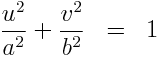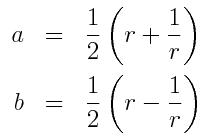# Joukowsky transformation

The Joukowsky transformation, or Joukowsky map, is a simple function that comes up in aerospace and electrical engineering calculations.(Here z is a complex variable.) Despite its simplicity, it’s interesting to look at in detail.## Mapping lines and circles

Let zr exp(iθ) and let w = uiv be its image. Writing the Joukowsky transformation in terms of its real and complex parts makes it easier to understand how it transforms lines and circles.We can see how circles are mapped by holding r constant and letting θ vary. The unit circle gets crushed to the interval [-1, 1] on the real axis, traversed twice. Circles of radius ρ ≠ 1 get mapped to ellipseswhereNext we hold θ constant and let r vary. If sin θ = 0 and cos θ > 0 then the image is [1, ∞). If sin θ = 0 and cos θ < 0 then the image is (-∞, -1]. In both cases the image is traced out twice. The image of the line with cos θ = 0 is the vertical axis. Otherwise lines through the origin are mapped to hyperbolas with equation## Inverse functions

If (z + 1/z)/2 = w then z2 -2wz + 1 = 0. The discriminant of this equation is 4(w2 – 1) and so the Joukowsky transformation is 2-to-1 unless w = ± 1, in which case z = ± 1. The product of two roots of a quadratic equation equals the constant term divided by the leading coefficient. In this case, the product is 1. This says the Joukowski transformation is 1-to-1 in any region that doesn’t contain both z and 1/z. This is the case for the interior or exterior of the unit circle, or of the upper or lower half planes. In any of those four regions, one can invert the Joukowski transformation by solving a quadratic equation and choosing the correct root.

Read more: Applied complex analysis

## 2 thoughts on “Joukowsky transformation”

1. Hossein Eskandari

Hi

I think this mapping transform the exterior parts to each other. Am I correct?

If so, is there any mapping to transform the interior of a circle to the interior of an ellipse?